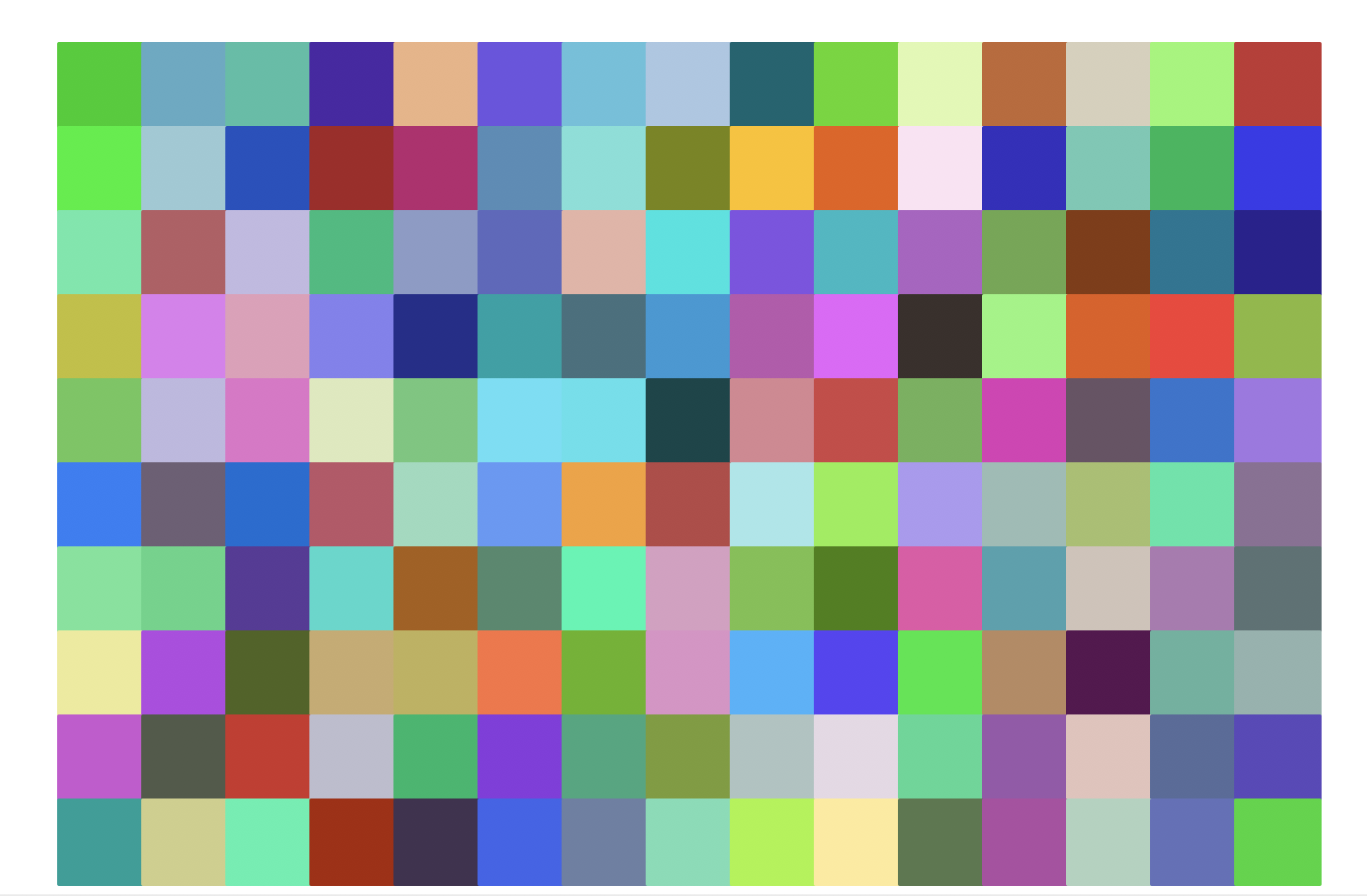Lab 6, Part 2: Turtle Graphics with For Loops

This part of today's lab should be completed in the provided lab06/grids.py file.

In this task, you'll complete a function called randomSquares that draws random square on the canvas. Below two different invocations are shown on the same canvas:

randomSquares(10, 50, 'red')  # 10 red randomly-positioned squares of size 50
randomSquares(20, 10, 'cyan') # 20 cyan randomly-positioned squares of size 10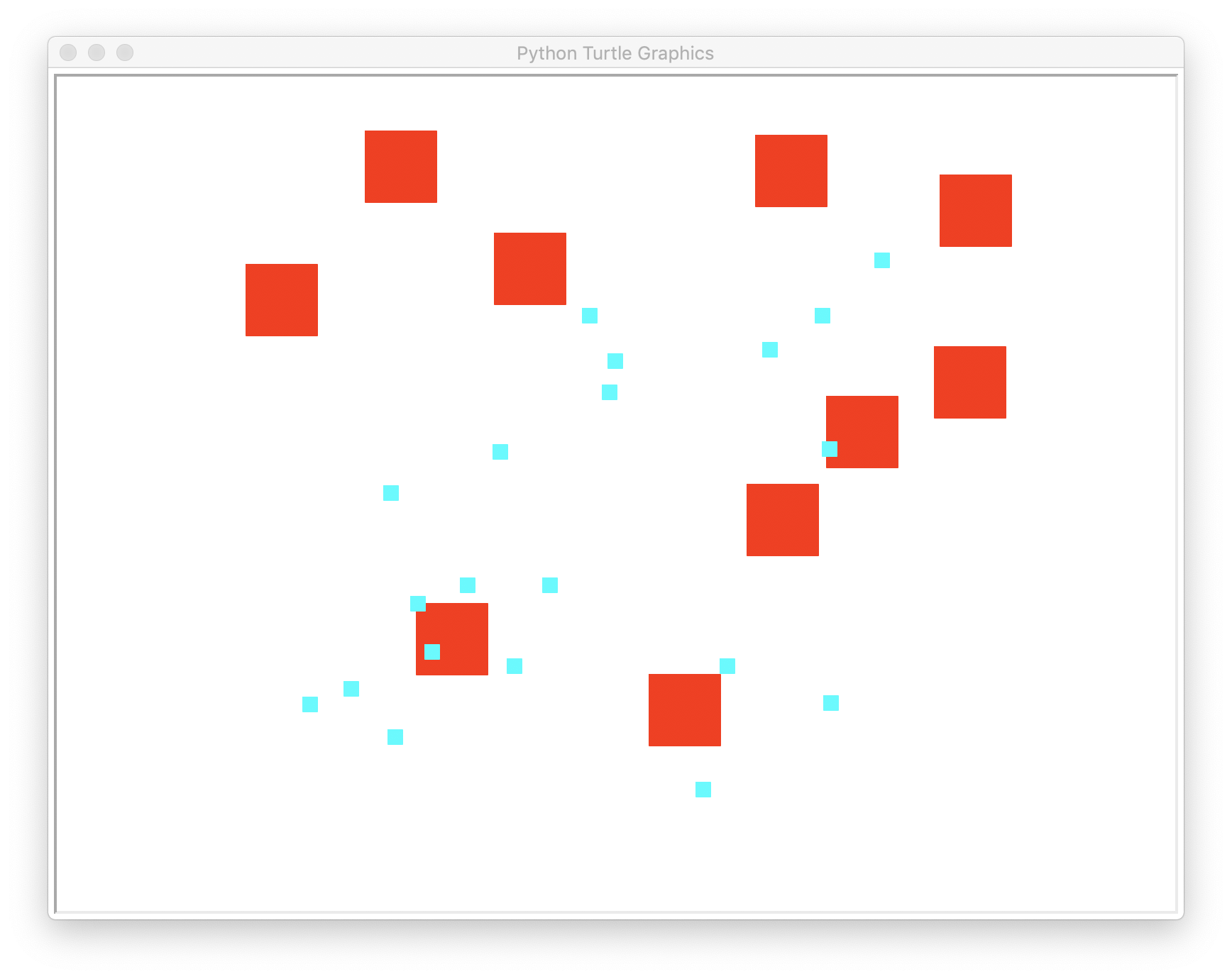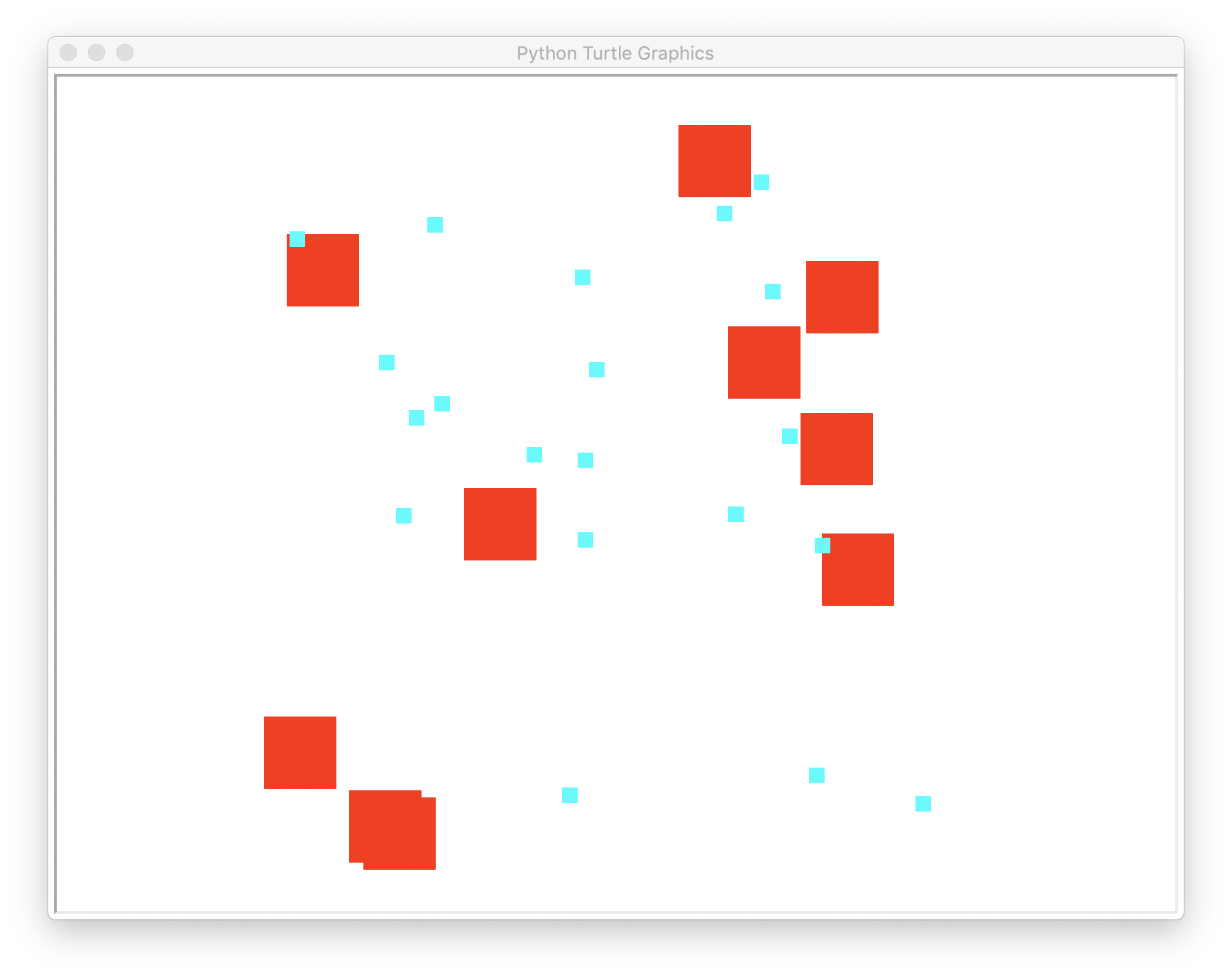Your randomSquares function should take the following 3 parameters:

1. number of squares in the Layer
2. size of the individual squares
3. color of the squares

Each time through the loop, one square is drawn. The turtle is repositioned before drawing each square at a randomly generated location (the x coordinate of the center point is a random integer between -400 and 400, and the same is true for the y coordinate of the center point).

Hint: use random.randint(). Here are two randint refresher links: last week's lab with the coin flip & general python documentation pages for random numbers.

Develop your code in baby steps. Write your function, at first, to simply produce the right number of squares. After that works, then work on placing them in random locations.

Tips:

1. Each time through the loop, you will draw another square
2. The function is not fruitful; it merely draws
3. Since the points are random, your results will differ from what is depicted above

Here is how you can test your randomSquares function:

if __name__ == "__main__":

#                         replace these with actual values
randomSquares(HOW_MANY, SIZE, COLOR)

Note that the above testing code should be placed in the __name__ == "__main__" conditional found in lab06/grids.py; you can assume all upcoming test invocations should also go in this main conditional. Ref: Disabling test work)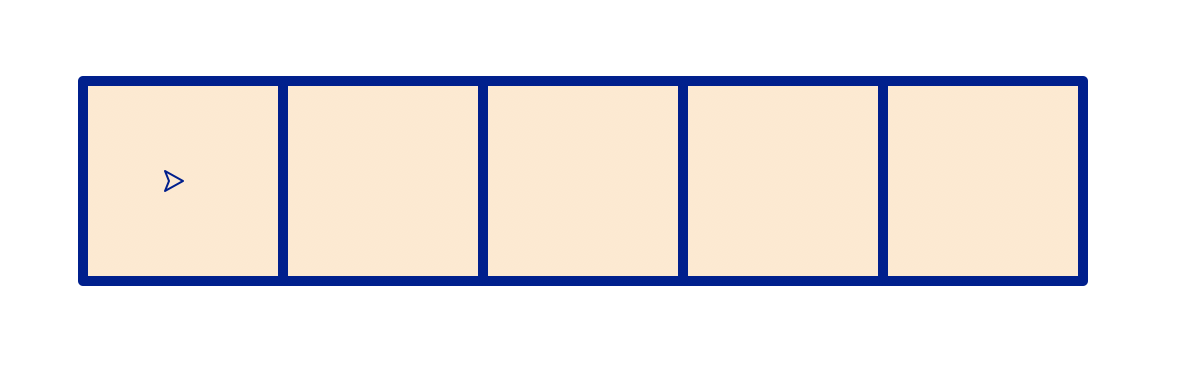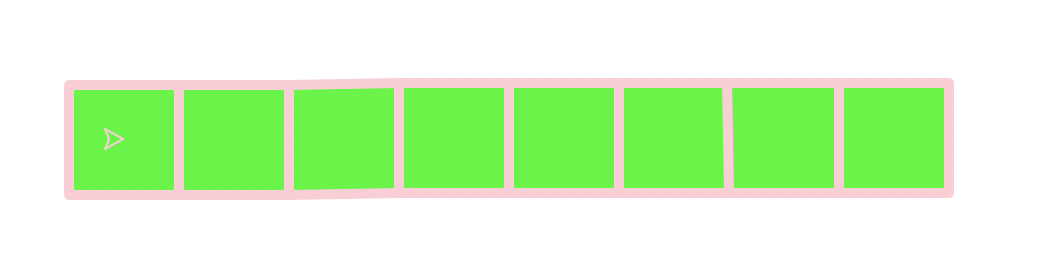In this task, you'll complete a function called makeRow that draws a row of squares.

This function should take the following 4 parameters:

1. number of squares in the row
2. size of the individual squares
3. color of the outline of the squares
4. color of the fill of the squares

The row is produced by adding one new square each time through the loop.

Tips:

1. Each time through the loop, you will add draw another square
2. Each successive square's center point shifts size to the right
3. The function is not fruitful; it merely draws
4. The function maintains a heading and position invariant (so the turtle ends up where it started when the function is complete)

Here is how you can test your makeRow function:

if __name__ == "__main__":

makeRow(5, 100, 'navy', 'bisque')
makeRow(8, 55, 'pink', 'green')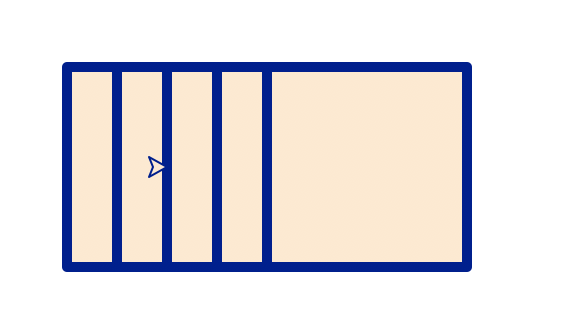Create a new function called makeOverlapRow that draws a row of overlapping squares.

This function takes 4 parameters:

1. the number of squares
2. the size of the square
3. the color of the outline of the square
4. the color of the interior of the square

Tips:

1. Each time through the loop, you will add draw another square
2. Each successive square's center point shifts size/4 to the right
3. The function is not fruitful; it merely draws
4. The function maintains a heading and position invariant (so the turtle ends up where it started when the function is complete)

Starting testing code (needs to be completed):

# [your code here] # Draw the overlapping row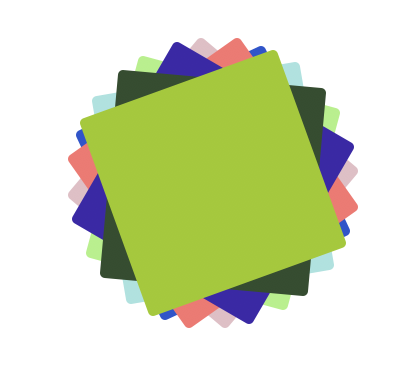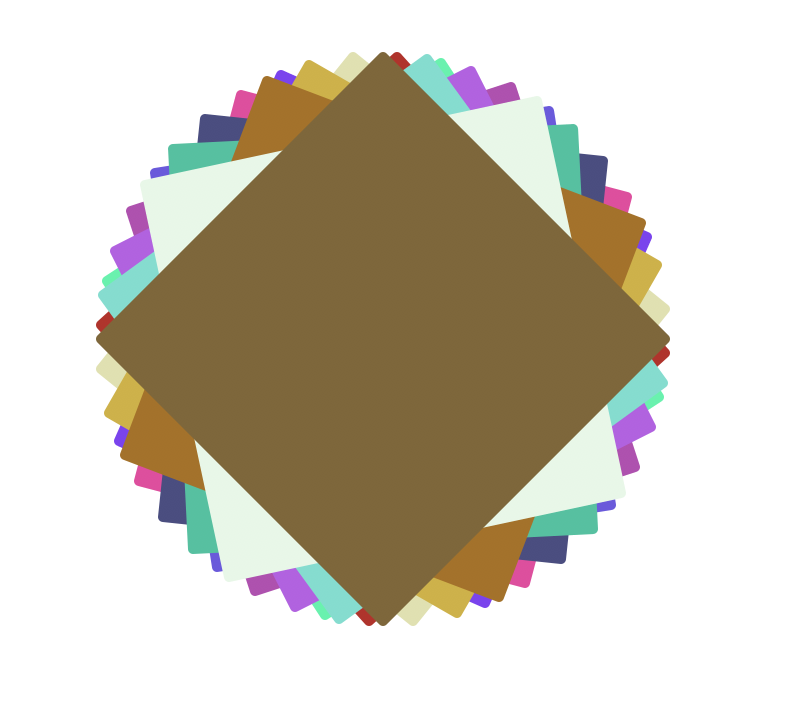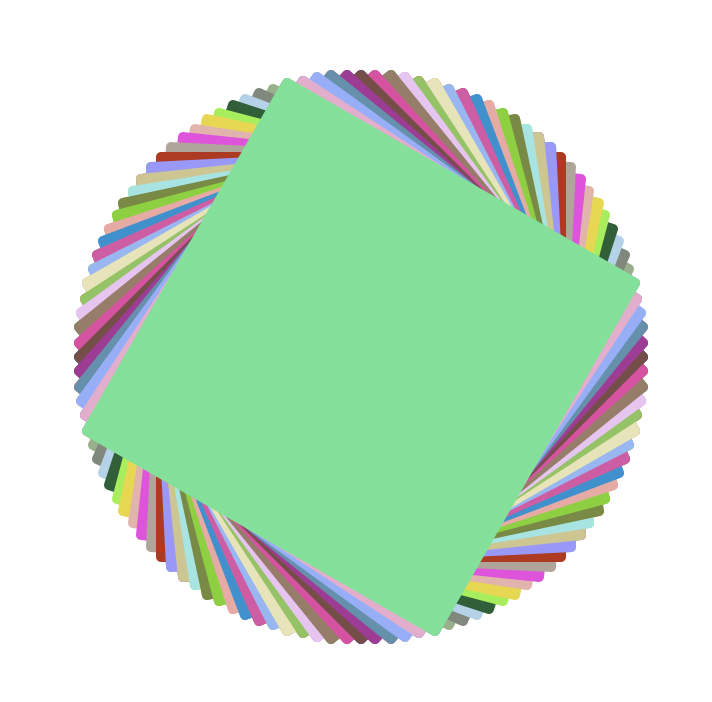Create a new function called makeTwistySquares that draws a set of overlapping rotated squares of varying colors.

This function should take the following 3 parameters:

1. number of squares
2. size of the square
3. angle of rotation

Like the previous function, colors should randomly be generated for each square.

Tips:

1. Each time through the loop, you will draw another square
2. Each successive square rotates angle degrees more than its predecessor
3. Use the provided randomTurtleColor() function to generate a random color for each square drawn, or use one of the random color functions found in turtleBeads.py, like randomPastelColor or randomWarmColor.
4. The function is not fruitful; it merely draws
5. The function maintains a heading and position invariant (so the turtle ends up where it started when the function is complete)

Testing code (the images above were generated with the following invocations, each on a new canvas):

makeTwistySquares(8, 100, 25)
makeTwistySquares(15, 200, 33)
makeTwistySquares(50, 200, 3)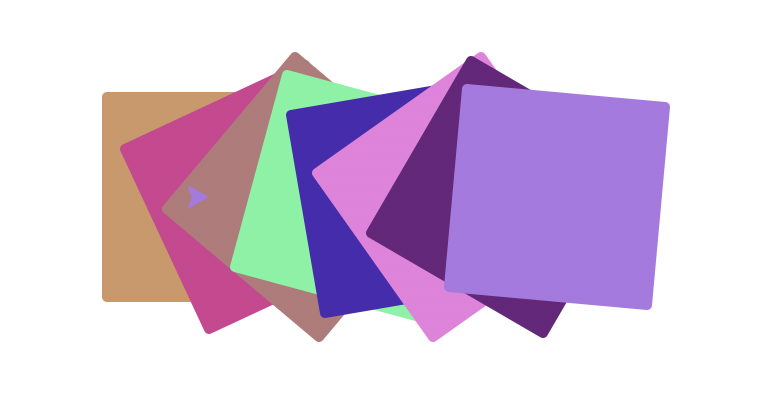Create a new function called makeRotatedRow that draws a row of rotated squares of varying colors.

This function takes the following 3 parameters:

1. number of squares
2. size of squares
3. angle of rotation

Note there is no color parameter; each square's color should be randomly generated using the provided function randomTurtleColor(), or one of the random color functions from turtleBeads; example usage:

# sets variable randomColor to store a randomly generated color
randomColor = randomTurtleColor()
# picks from a specific set of warm colors (see turtleBeasd.py)
randomColor = randomWarmColor()

(randomTurtleColor works by randomly generating three integers between [0, 1] that are the Red, Green and Blue values, respectively. You can read more about RGB colors here)

Tips:

1. Each time through the loop you will draw another square
2. Each successive square's center point shifts size/4 to the right
3. Each successive square rotates angle degrees more than its predecessor
4. The function is not fruitful; it merely draws
5. The function maintains a heading and position invariant (so the turtle ends up where it started when the function is complete)

Create a new function called makeGrid that draws a set of squares arranged in a circle-like shape, with each corner touching the corner of the two adjacent squares. This function takes the following parameters:

1. Number of squares (petals)
2. size of each square
3. color of all the squares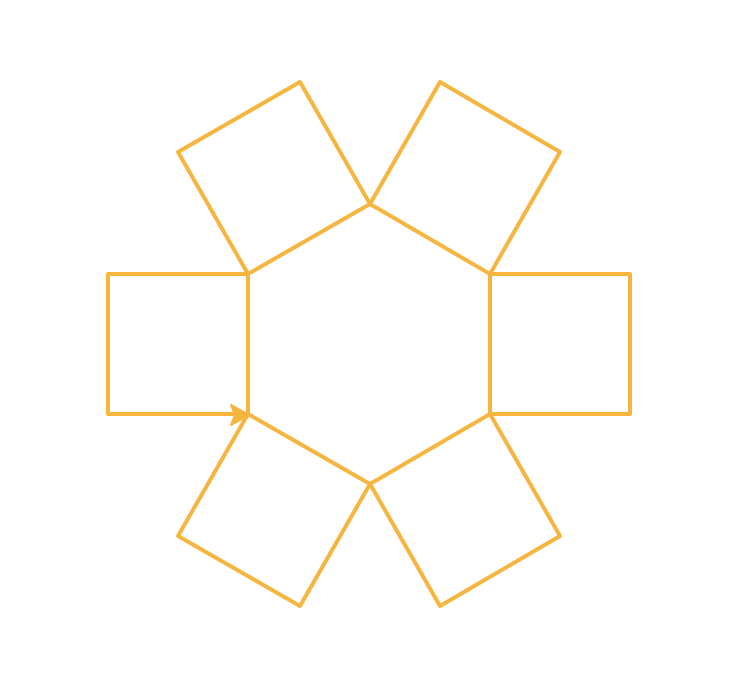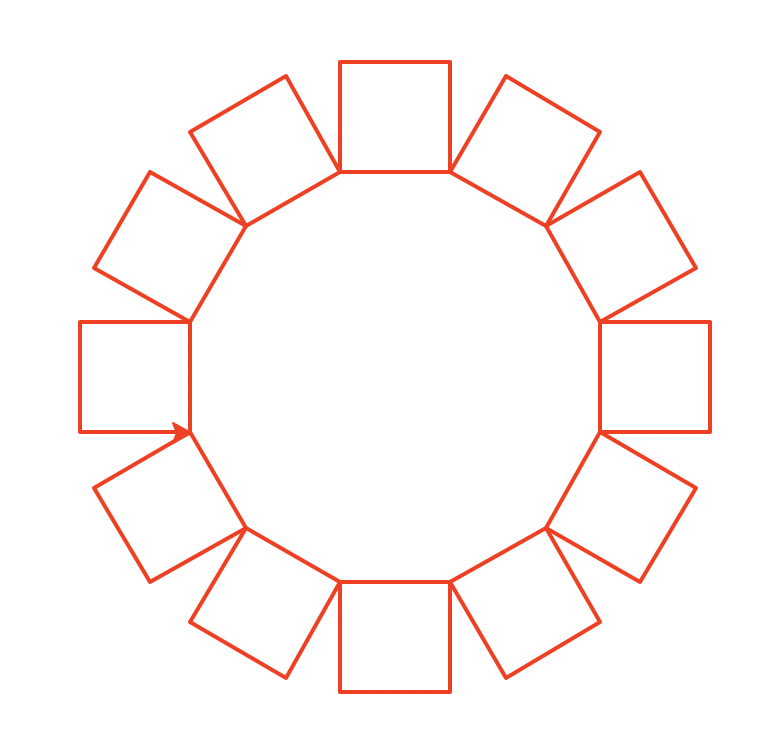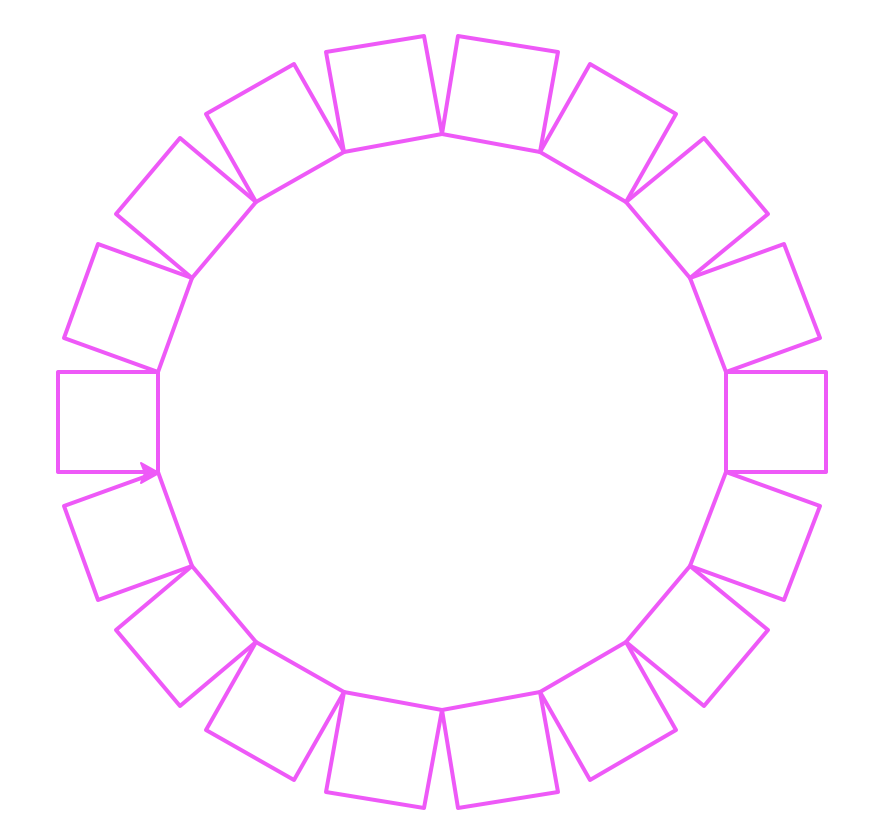Tips:

1. Each time through the loop, you will draw another square
2. You can divide 360/number of squares to determine the angle of rotation
3. The function is not fruitful; it merely draws
4. The function maintains a heading and position invariant (so the turtle ends up where it started when the function is complete). In this case, the turtle starts and finishes at the lower right corner of the leftmost flower "petal". If you look carefully, you can see the turtle in each of the images above.

Examples:

makeFlower(6, 70, 'orange')
makeFlower(12, 55, 'red')
makeFlower(18, 50, 'magenta')

Create a new function called makeGrid that draws a set of squares arranged in a grid. This function takes the following parameters:

1. rows in the grid
2. columns in the grid
3. The size of each square
4. color1 outer square color
5. color2 fill square color

Examples:

makeGrid(8, 3, 50, 'orange', 'grey')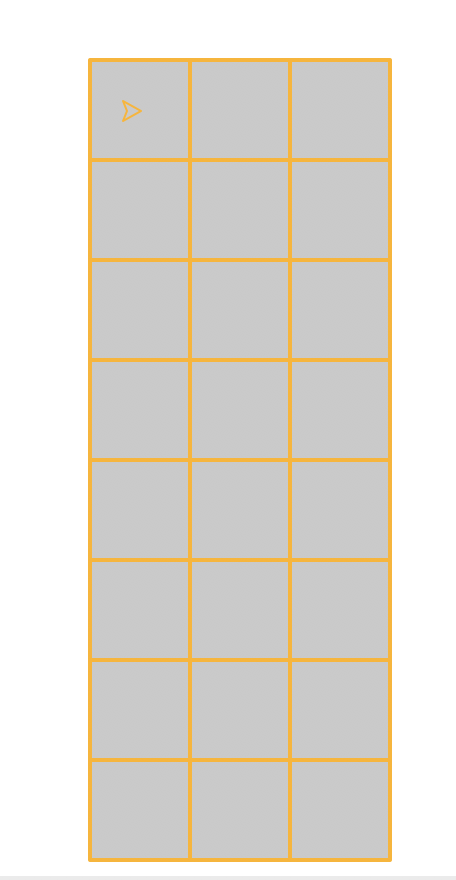makeGrid(4, 10, 50, 'gray', 'black')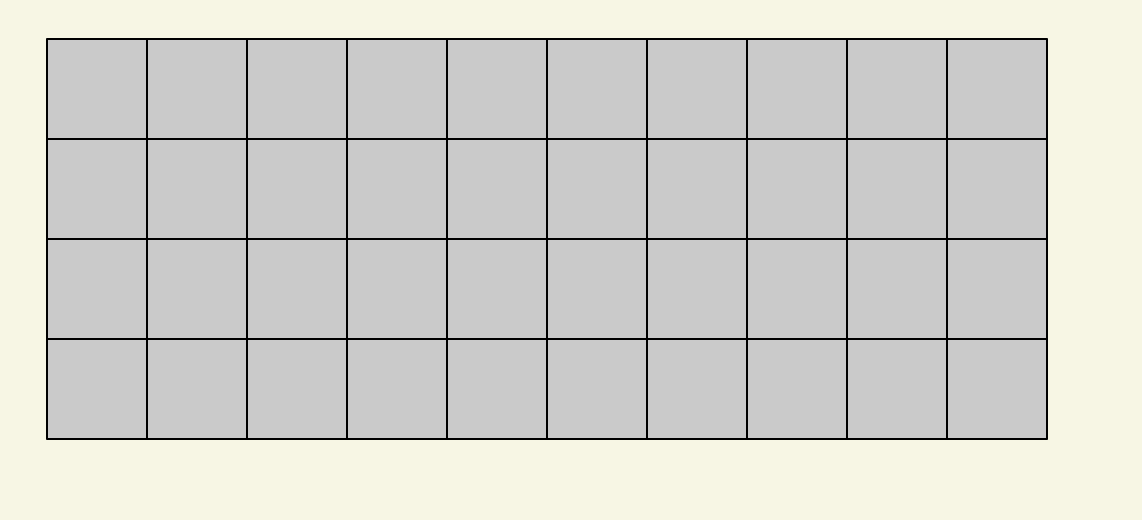makeGrid(2, 15, 30, 'black', 'orange')

<img src="orange.png" style="width:300px" alt="A 15-column 2-row grid with orange squares."

The grids can be large, so make sure to start with a fresh canvas for each one.

Tips:

1. You can use a nested loop and draw squares, or you can use a loop that invokes makeRow
2. The function is not fruitful; it merely draws
3. Make sure you test your function on a variety of different sized grids

Create a new function named makeRowColorGrid that draws squares arranged in a grid, where each row of squares is a random color.

This function may, but does not need to, use the makeRow function you've already created.

This function should take the following 3 parameters:

1. Number of rows
2. Number of columns
3. size of the individual squares

Example

makeRowColorGrid(8, 3, 50)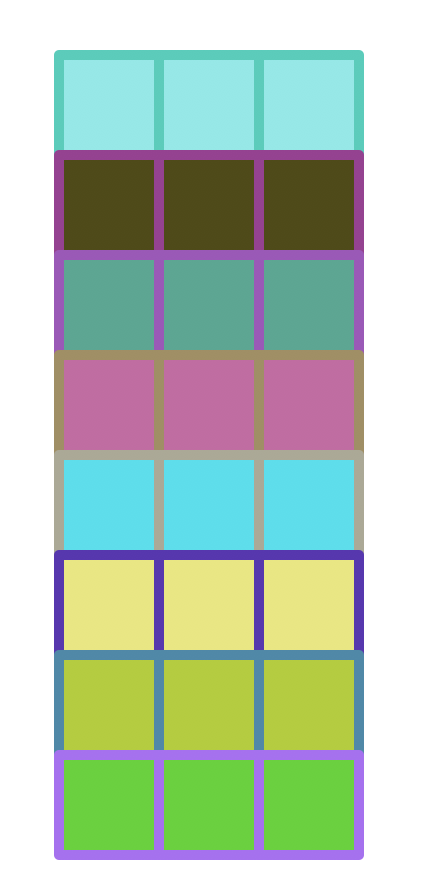Create a new function named makeRandomColorGrid that draws squares arranged in a grid, where each row of squares is a random color.

This function should take the following 3 parameters:

1. Number of rows
2. Number of columns
3. size of the individual squares

Each square has a randomly assigned color (use the provided randomTurtleColor() function).

makeRandomColorGrid(10, 15, 50)